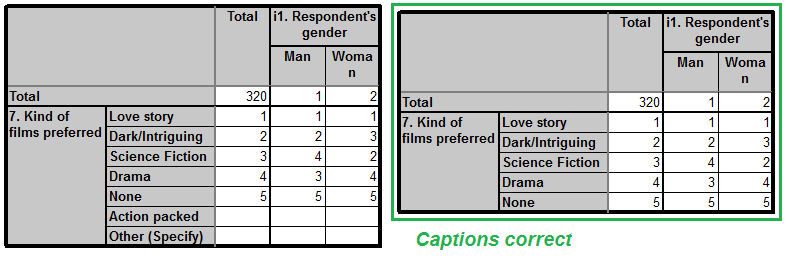Scroll

# Ranking Table

Follow
 Summary This article is one in a series of articles that will go through examples of using table arithmetic syntax new to Askia 5.3.5.0. This article demonstrates the post processing or 'Cleaning script' and calculation arithmetics. Applies to Askia Analyse. Written for Researchers, Developers, Data Processors. Keywords Post processing, Cleaning script, Format, Layout, Colour, Conditional formatting, Table arithmetic, Forloops, Coordinates, data, Calculation arithmetic, Rank, Ranked table, Top mentions.

The .qes file and portfolio containing the examples discussed is attached: Table Arithmetic Examples 3.rar

Firstly we will show how to use table arithmetic to delete the text in a table after a given row. Then we will show how to use the syntax to fill from one table into another. An example of when this could be useful is if you wanted to create a table that isolates and shows only the top ranked items in a table.

On the Ranked Original tab definition you’ll see we have one calculation set up to show the rank of the items.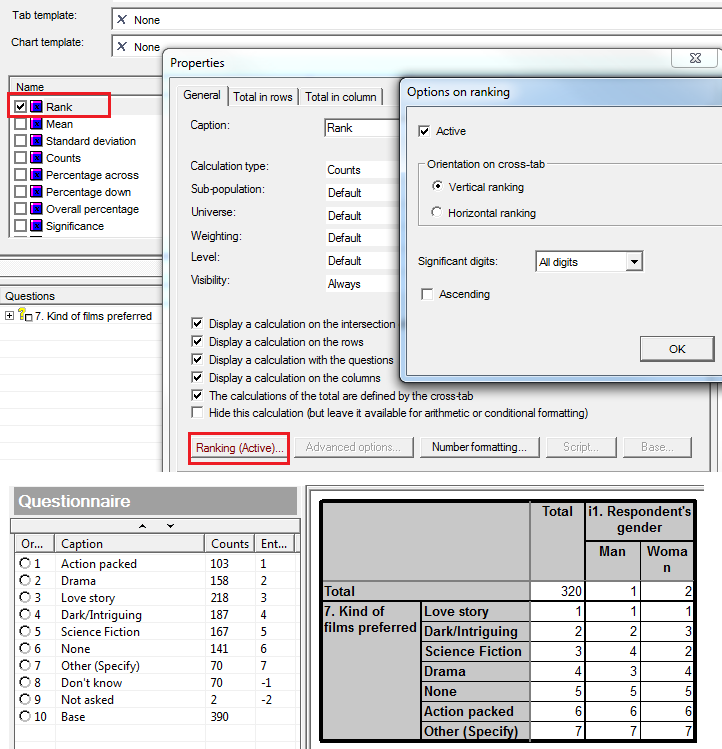In Rank Cleaned we have applied some cleaning script in the General tab > Settings > Sorting > Cleaning script.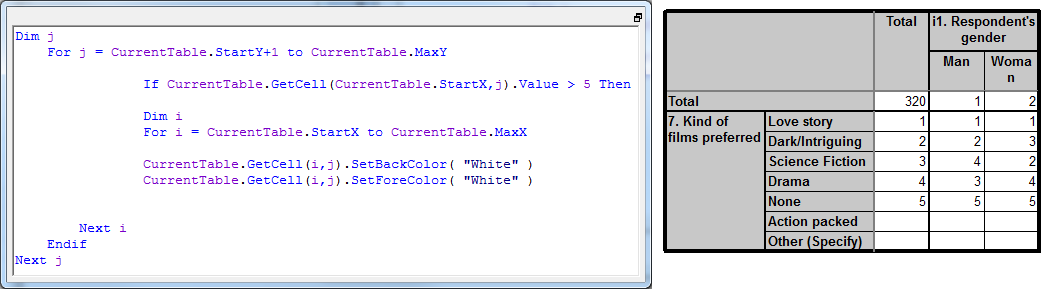You will notice the tab definition is a dark grey colour which means it won’t be output with the results. It’s only used as an intermediate table.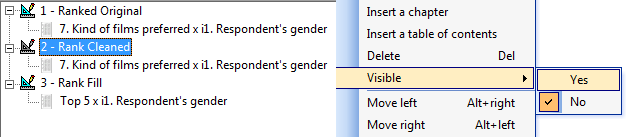In the final table Rank Fill we have created a dummy question called Top 5. The purpose of this is to simply show how we can push data into any variable structure we have created.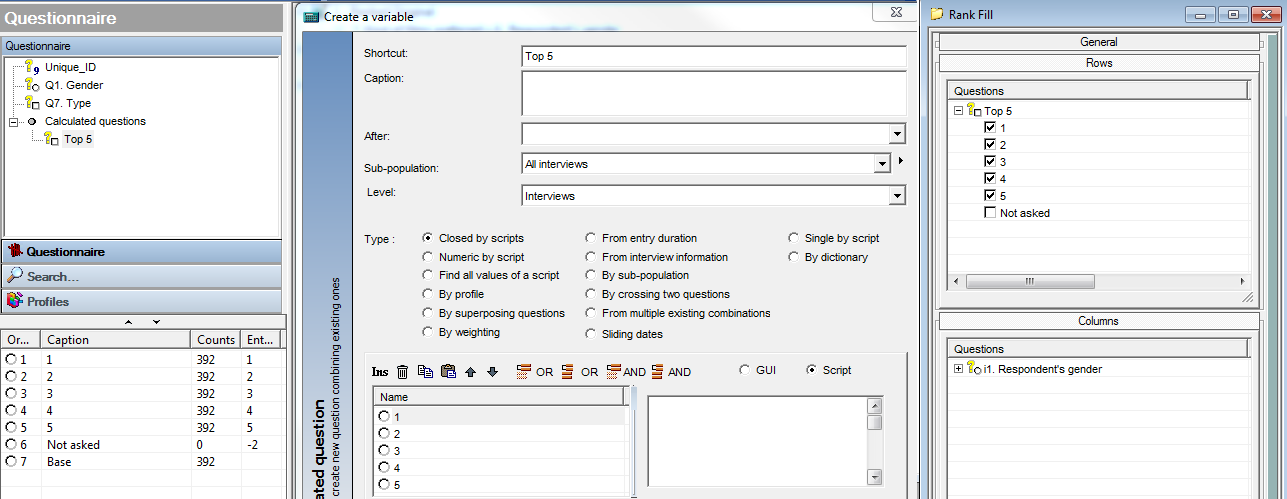Then in this table we insert a calculation arithmetic calculation and use the GetTable method as shown below to push the data into this table.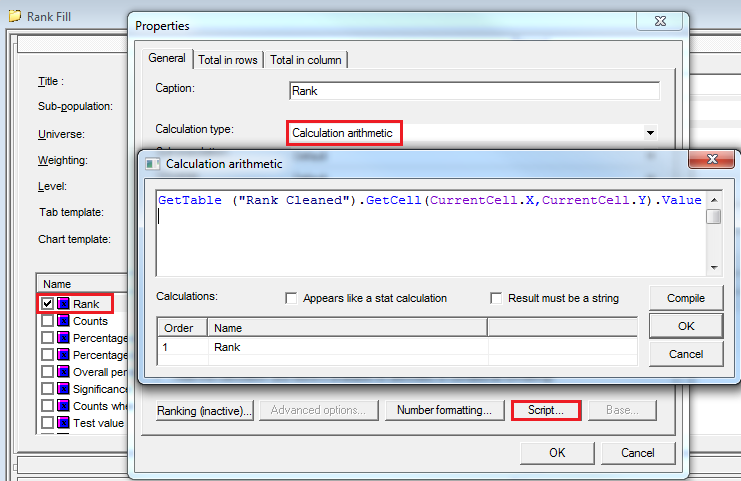This gives the following (right) compared to the original: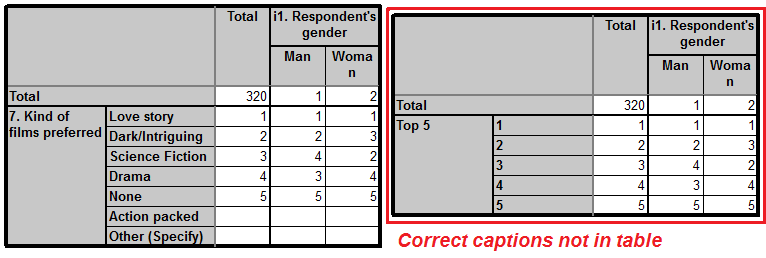In order to fill the captions of the first 5 codes from our source table we need to use cleaning script again and employ the SetText method.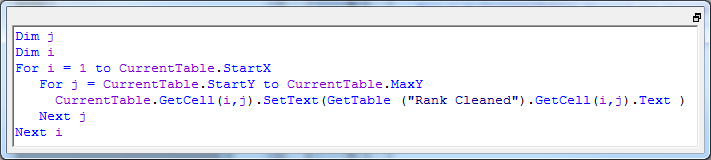The final result (right) compared to the original: